Next: Sample session with cdd+ Up: Voronoi Diagram and Delaunay Previous: Is it possible to   Contents

## Is it possible to determine the Delaunay cell containing a given point efficiently?

Yes, it is possible to find the nearest point set associated with the Delaunay cell containing a given point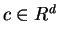. As we discussed in Section 3.3, the Delaunay complex can be represented by the convex hull of appropriately lifted points in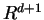, and the non-vertical facets coincide with the Delaunay cells once they are projected to the original space. Thus the problem of determining the Delaunay cell containing a given point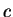can be reduced to finding the first facet of a polyhedron shoot'' by a ray.

To be more precise, let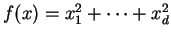, and let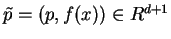for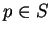. Then the lower hull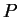of the lifted points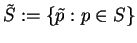: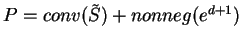represents the Delaunay complex. Here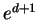is the unit vetor inwhose last component is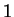. For any vectorand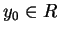, let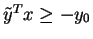denote a general inequality of a variable vector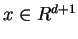. For such an inequality to represent a valid inequality of(see Section 2.2), it must be satisfied by all points in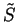: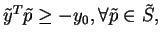(9)

and by any points shifted vertically upwards, i.e.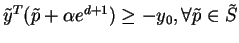and anyUnder the first inequality (9), the last inequality is equivalent to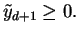(10)

Now every Delaunay cell is a projection of a non-vertical facet of. We are thus looking for an inequalitysatisfying (9), (10) and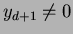. By scaling with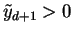, we may assume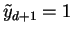. For a given point, let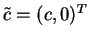, and let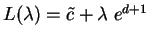,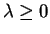. Determining the Delaunay cell containingis equivalent to finding the last inequality hit'' by the halfline. More precisely, it is to find a non-vertical facet inequality such that the intersecion point of the corresponding hyperplane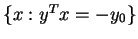and the half line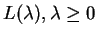is highest possible.

By substituting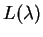for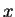in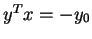with, we obtain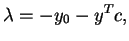where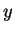denotes the vector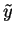without the last coordinate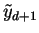. The LP formulation is therefore:

 minimize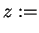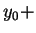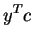(11) subject to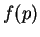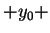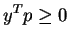for all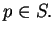While an optimal solution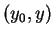to this LP may not determine any facet in general, the simplex method always returns an optimal basic solution which determines a facet inequality in this case. The Delaunay cell containingis the one determined by the set of points in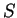whose corresponding inequalities are satisfied by equality at the optimal solution. If the LP solution is not degenerate, the dual variables that are positive at the dual optimal solution coincides with the former set.

It is important to note that the above LP might be unbounded. If it is unbounded, it can be easily shown thatis not in any Delaunay cell, i.e., not in the convex hull of. A certificate of unboundedness actually induces a hyperplane strongly separatingfrom. (why?)

SubsectionsNext: Sample session with cdd+ Up: Voronoi Diagram and Delaunay Previous: Is it possible to   Contents
Komei Fukuda 2004-08-26